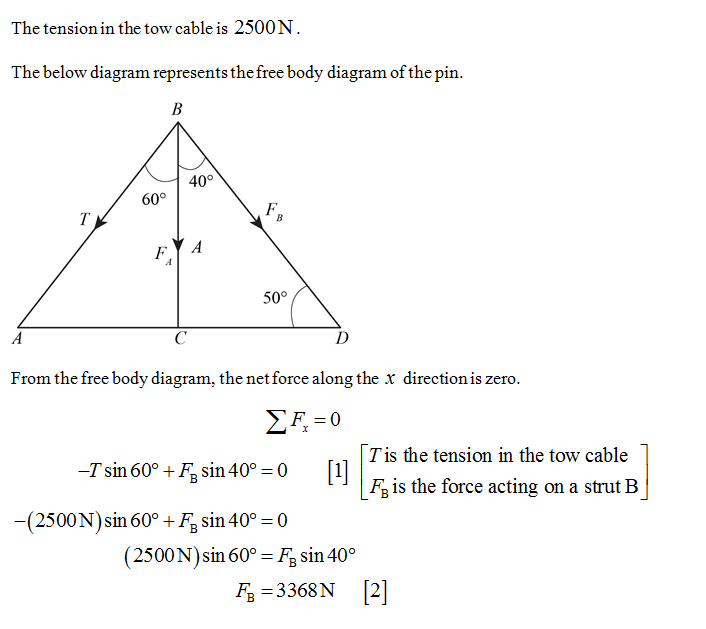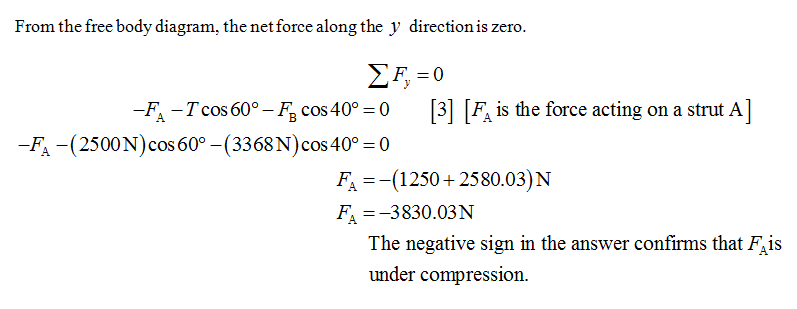# A car is stuck in the mud. A tow truck pulls on the car with the arrangement shown in Figure P5.24. The tow cable is under a tension of 2500 N and pulls downward and to the left on the pin at its upper end. The light pin is held in equilibrium by forces exerted by the two bars A and B. Each bar is a strut; that is, each is a bar whose weight is small compared to the forces it exerts and which exerts forces only through hinge pins at its ends. Each strut exerts a force directed parallel to its length. Determine the force of tension or compression in each strut. Proceed as follows. Make a guess as to which way (pushing or pulling) each force acts on the top pin. Draw a free-body diagram of the pin. Use the condition for equilibrium of the pin to translate the free-body diagram into equations. From the equations calculate the forces exerted by struts A and B. If you obtain a positive answer, you correctly guessed the direction of the force. A negative answer means that the direction should be reversed, but the absolute value correctly gives the magnitude of the force. If a strut pulls on a pin, it is in tension. If it pushes, the strut is in compression. Identify whether each strut is in tension or in compression.

Question
4 views

A car is stuck in the mud. A tow truck pulls on the car with the arrangement shown in Figure P5.24. The tow cable is under a tension of 2500 N and pulls downward and to the left on the pin at its upper end. The light pin is held in equilibrium by forces exerted by the two bars A and B. Each bar is a strut; that is, each is a bar whose weight is small compared to the forces it exerts and which exerts forces only through hinge pins at its ends. Each strut exerts a force directed parallel to its length. Determine the force of tension or compression in each strut. Proceed as follows. Make a guess as to which way (pushing or pulling) each force acts on the top pin. Draw a free-body diagram of the pin. Use the condition for equilibrium of the pin to translate the free-body diagram into equations. From the equations calculate the forces exerted by struts A and B. If you obtain a positive answer, you correctly guessed the direction of the force. A negative answer means that the direction should be reversed, but the absolute value correctly gives the magnitude of the force. If a strut pulls on a pin, it is in tension. If it pushes, the strut is in compression. Identify whether each strut is in tension or in compression.

check_circle

Step 1Step 2...

### Want to see the full answer?

See Solution

#### Want to see this answer and more?

Solutions are written by subject experts who are available 24/7. Questions are typically answered within 1 hour.*

See Solution
*Response times may vary by subject and question.
Tagged in